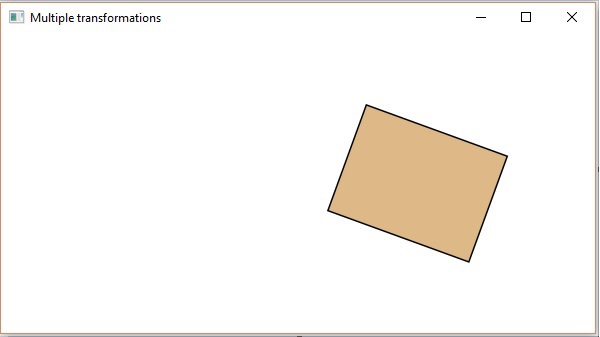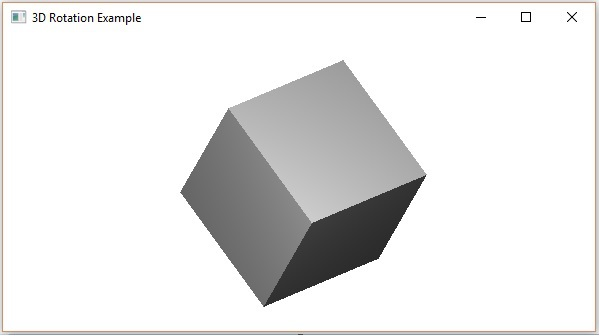# JavaFX - Transformations

Transformation means changing some graphics into something else by applying rules. We can have various types of transformations such as Translation, Scaling Up or Down, Rotation, Shearing, etc.

Using JavaFX, you can apply transformations on nodes such as rotation, scaling and translation. All these transformations are represented by various classes and these belong to the package javafx.scene.transform.

S.No Transformation & Description
1 Rotation

In rotation, we rotate the object at a particular angle θ (theta) from its origin.

2 Scaling

To change the size of an object, scaling transformation is used.

3 Translation

Moves an object to a different position on the screen.

4 Shearing

A transformation that slants the shape of an object is called the Shear Transformation.

## Multiple Transformations

You can also apply multiple transformations on nodes in JavaFX. The following program is an example which performs Rotation, Scaling and Translation transformations on a rectangle simultaneously.

Save this code in a file with the name −

MultipleTransformationsExample.java.

```import javafx.application.Application;
import javafx.scene.Group;
import javafx.scene.Scene;
import javafx.scene.paint.Color;
import javafx.scene.shape.Rectangle;
import javafx.scene.transform.Rotate;
import javafx.scene.transform.Scale;
import javafx.scene.transform.Translate;
import javafx.stage.Stage;

public class MultipleTransformationsExample extends Application {
@Override
public void start(Stage stage) {
//Drawing a Rectangle
Rectangle rectangle = new Rectangle(50, 50, 100, 75);

//Setting the color of the rectangle
rectangle.setFill(Color.BURLYWOOD);

//Setting the stroke color of the rectangle
rectangle.setStroke(Color.BLACK);

//creating the rotation transformation
Rotate rotate = new Rotate();

//Setting the angle for the rotation
rotate.setAngle(20);

//Setting pivot points for the rotation
rotate.setPivotX(150);
rotate.setPivotY(225);

//Creating the scale transformation
Scale scale = new Scale();

//Setting the dimensions for the transformation
scale.setX(1.5);
scale.setY(1.5);

//Setting the pivot point for the transformation
scale.setPivotX(300);
scale.setPivotY(135);

//Creating the translation transformation
Translate translate = new Translate();

//Setting the X,Y,Z coordinates to apply the translation
translate.setX(250);
translate.setY(0);
translate.setZ(0);

//Adding all the transformations to the rectangle

//Creating a Group object
Group root = new Group(rectangle);

//Creating a scene object
Scene scene = new Scene(root, 600, 300);

//Setting title to the Stage
stage.setTitle("Multiple transformations");

stage.setScene(scene);

//Displaying the contents of the stage
stage.show();
}
public static void main(String args[]){
launch(args);
}
}
```

Compile and execute the saved java file from the command prompt using the following commands.

```javac MultipleTransformationsExample.java
java MultipleTransformationsExample
```

On executing, the above program generates a JavaFX window as shown below.## Transformations on 3D Objects

You can also apply transformations on 3D objects. Following is an example which rotates and translates a 3-Dimensional box.

Save this code in a file with the name RotationExample3D.java.

```import javafx.application.Application;
import javafx.scene.Group;
import javafx.scene.Scene;
import javafx.scene.shape.Box;
import javafx.scene.transform.Rotate;
import javafx.scene.transform.Translate;
import javafx.stage.Stage;

public class RotationExample3D extends Application {
@Override
public void start(Stage stage) {
//Drawing a Box
Box box = new Box();

//Setting the properties of the Box
box.setWidth(150.0);
box.setHeight(150.0);
box.setDepth(150.0);

//Creating the translation transformation
Translate translate = new Translate();
translate.setX(400);
translate.setY(150);
translate.setZ(25);

Rotate rxBox = new Rotate(0, 0, 0, 0, Rotate.X_AXIS);
Rotate ryBox = new Rotate(0, 0, 0, 0, Rotate.Y_AXIS);
Rotate rzBox = new Rotate(0, 0, 0, 0, Rotate.Z_AXIS);
rxBox.setAngle(30);
ryBox.setAngle(50);
rzBox.setAngle(30);

//Creating a Group object
Group root = new Group(box);

//Creating a scene object
Scene scene = new Scene(root, 600, 300);

//Setting title to the Stage
stage.setTitle("Drawing a cylinder");

stage.setScene(scene);

//Displaying the contents of the stage
stage.show();
}
public static void main(String args[]){
launch(args);
}
}
```

Compile and execute the saved java file from the command prompt using the following commands.

```javac RotationExample3D.java
java RotationExample3D
```

On executing, the above program generates a JavaFX window as shown below.